## Why to analyze?

To solve a single problem, there may be many different algorithms. Should we just randomly pick up one or use the best one? Of course, we want the best one. But how can we assert that this one is better than that one? Even though we have the best one, is it really the best one in the world? Can there be a better undiscovered algorithm?

We must analyze algorithms to:

• predict performance
• compare algorithms
• provide guarantees
• understand theoretical basis

## What to analyze?

This question is rather easy to answer. Since we use computer to run algorithms, we want to know:

• how long does one algorithm take
• how much memory does it need

## How to analyze?

This is the major question we want to answer today. To analyze algorithms, we must have a scientific method, a framwork to predict performance and compares algorithms:

• Observe some feature of the natural world
• Hypothesize a model that is consistent with the observations
• Predict events using the hypothesis
• Verify the predictions by making futher observations
• Validate by repeating until the hypothesis and observations agree

And there are two important priciples:

• Experiments  must be reproducible
• Hypothesis must be falsifiable

### Empirical Observation

Let’s take an example to analyze following the method above.

3-sum. Given N distinct integers, how many triples sum to exact zero?

 ``````1 2 3 4 5 `````` ``````% more 8ints.txt 8 30 -40 -20 -10 40 0 10 5 % java ThreeSum 8ints.txt 4 ``````

The most straightfoward algorithm is brute-force.

 `````` 1 2 3 4 5 6 7 8 9 10 11 12 13 14 15 16 `````` ``````public class ThreeSum{ public static int count(int[] a){ int N = a.length; int count = 0; for (int i = 0; i < N; i++) for (int j = i+1; j < N; j++) for (int k = j+1; k < N; k++) // check each triple if (a[i] + a[j] + a[k] == 0) count++; return count; } public static void main(String[] args){ int[] a = In.readInts(args); StdOut.println(count(a)); } } ``````

Run the program for various input sizes and measure running time. We get this: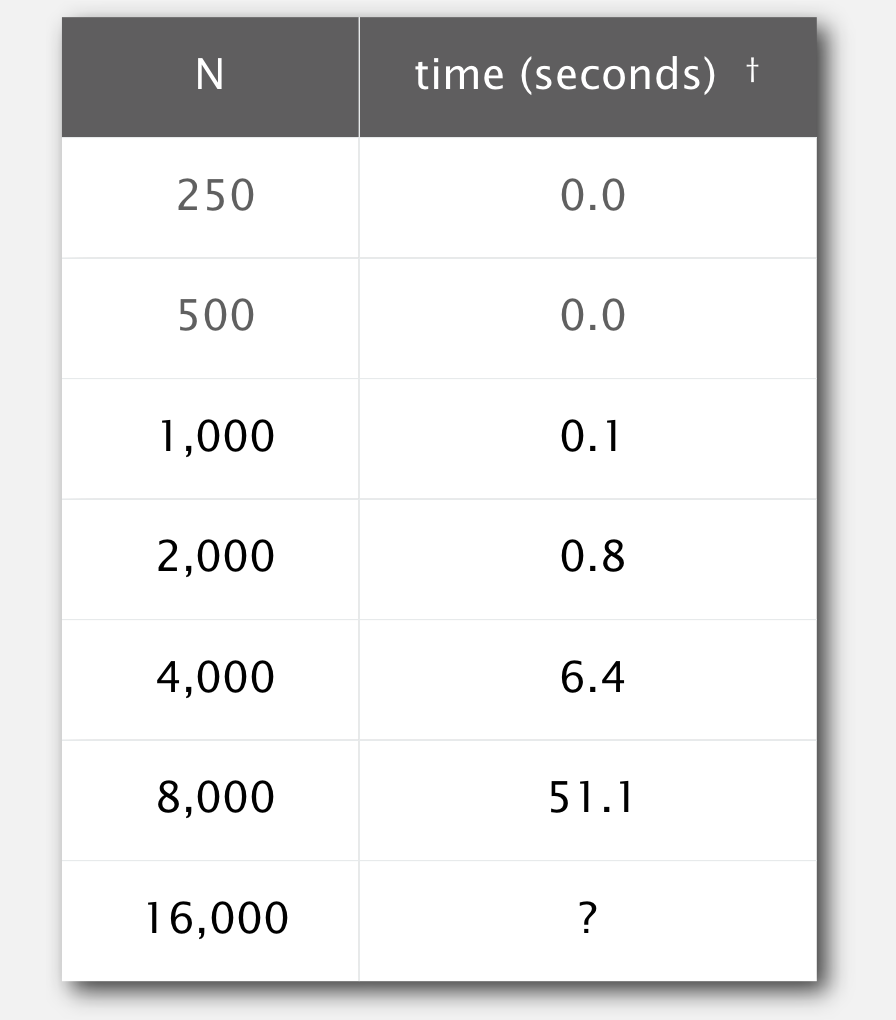We can draw a log-log plot.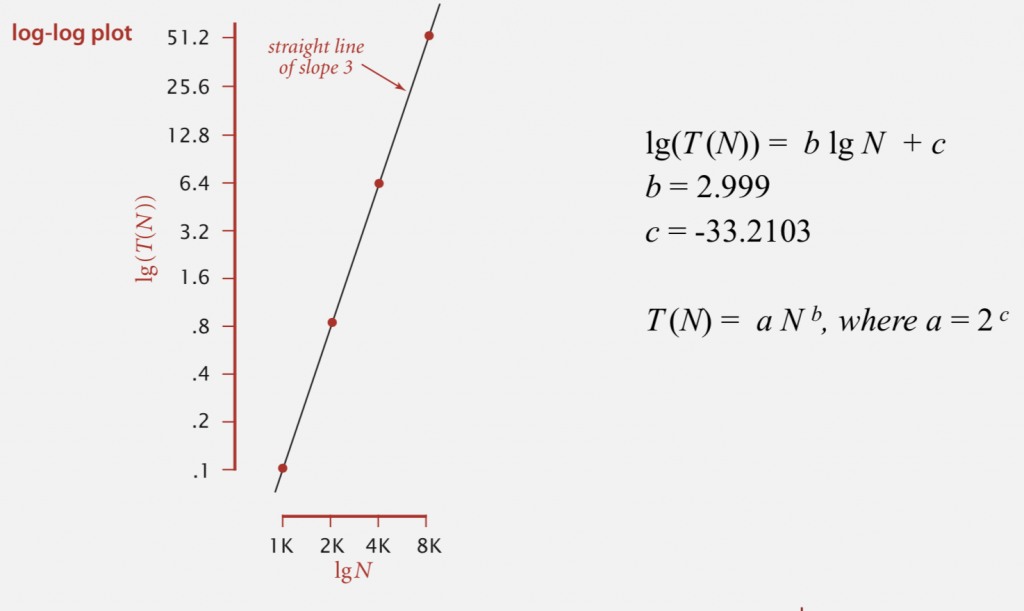Finally, we get a formula: T(N) = aNb. Here, we can have a hypothesis: The running time is about 1.006 × 10–10 × N2.999 seconds.

Since we have a hypothesis, we can make a prediction: the running time for N=16000 is 408.1. The actual running time is 410.8, which verifies our hypothesis. We can also check the table above to validate our hypothesis.

However, do we have to pick up a stop watch every time we want to analyze an algorithm? This is tedious, and there should be a cleverer way to do the job. Yes! There is!

### Mathematical model for running time

Total running time: sum of cost x frequency for all operations.

• Need to analyze program to determine set of operations
• Cost depends on machine, compiler.
• Frequency depends on algorithm, input data.

Here is a table of cost of basic operations: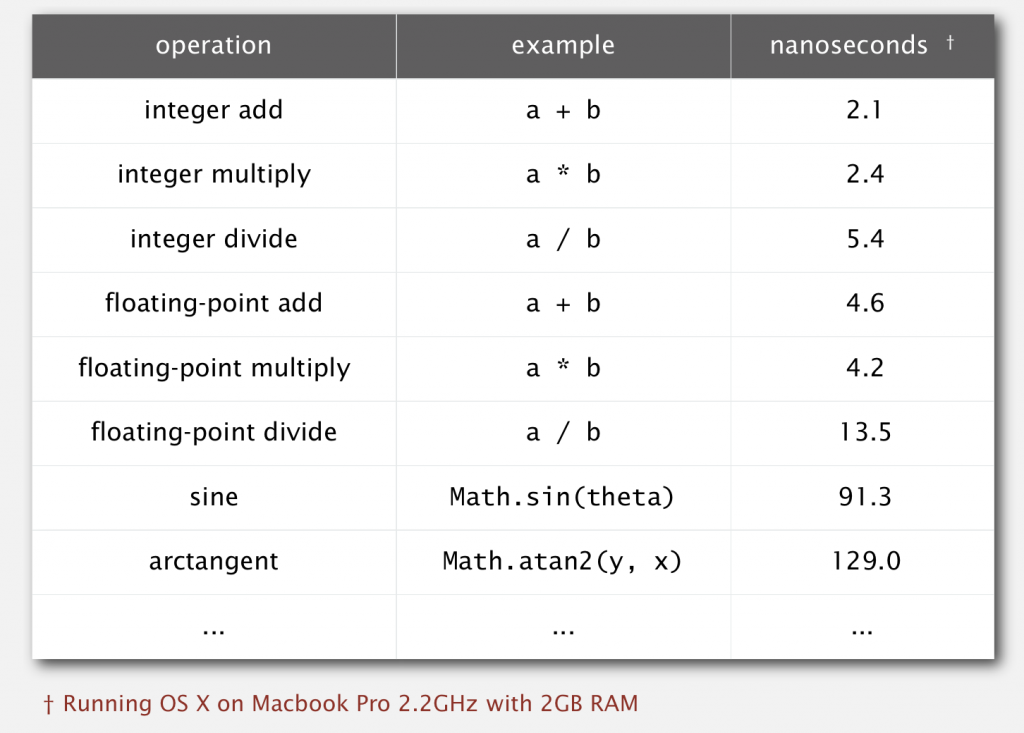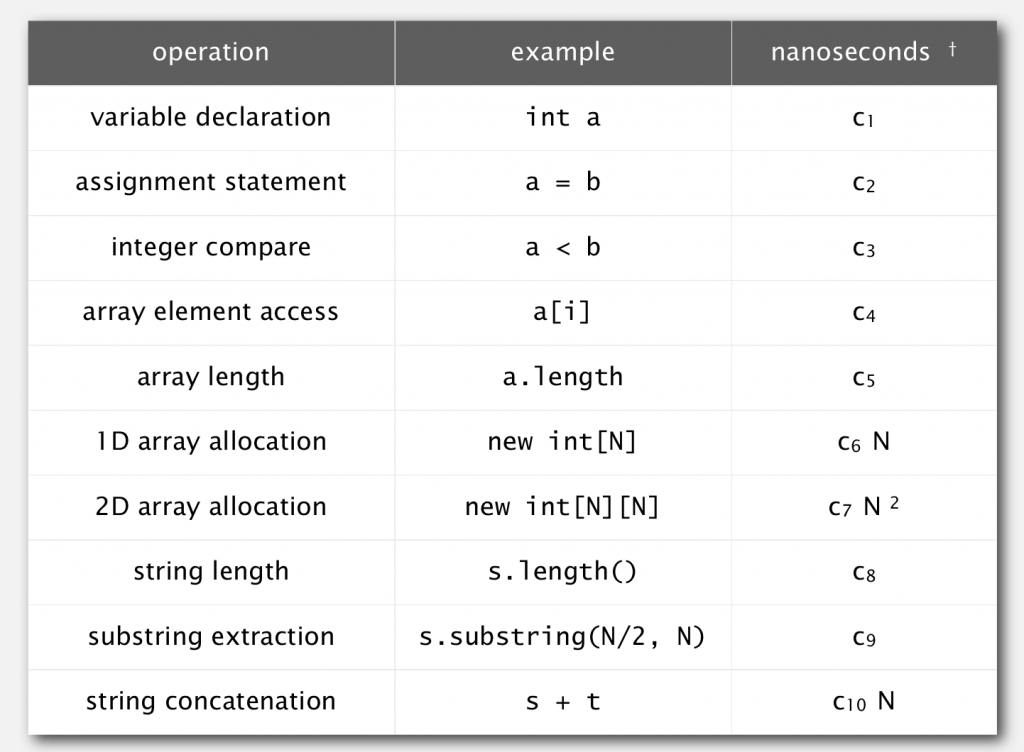So given the following code(2-sum):

 ``````1 2 3 4 5 `````` ``````int count = 0; for (int i = 0; i < N; i++) for (int j = i+1; j < N; j++) if (a[i] + a[j] == 0) count++; ``````

we have: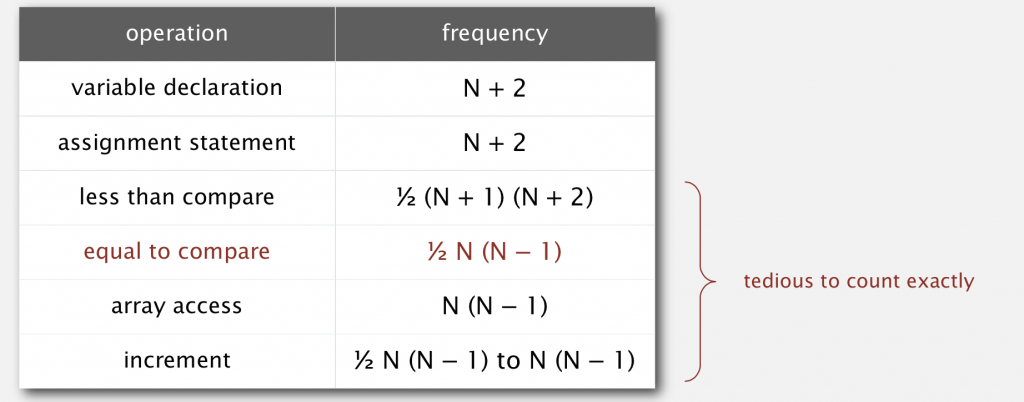Do we have to count these numbers up to get a exact number of running time? The answer is no. Instead of measuring exact time an algorithm takes, we count how many times basic operations(like array access) are done. And instead of using a formula like (1/6)N3 + 100N4/3 +56, we can use a tilde notation like ~(1/6)N3 by discarding lower-order terms, because as N goes larger and larger, lower-order terms make less sense to the result.

So the tilde notation of running time of code above is ~N2.

### Common order-of-growth classifications

A good news is that a small set of functions(1, logN, N, NlogN, N2, N3,and 2N) is sufficient to describe order-of-growth of typical algorithms. And here is a plot of them: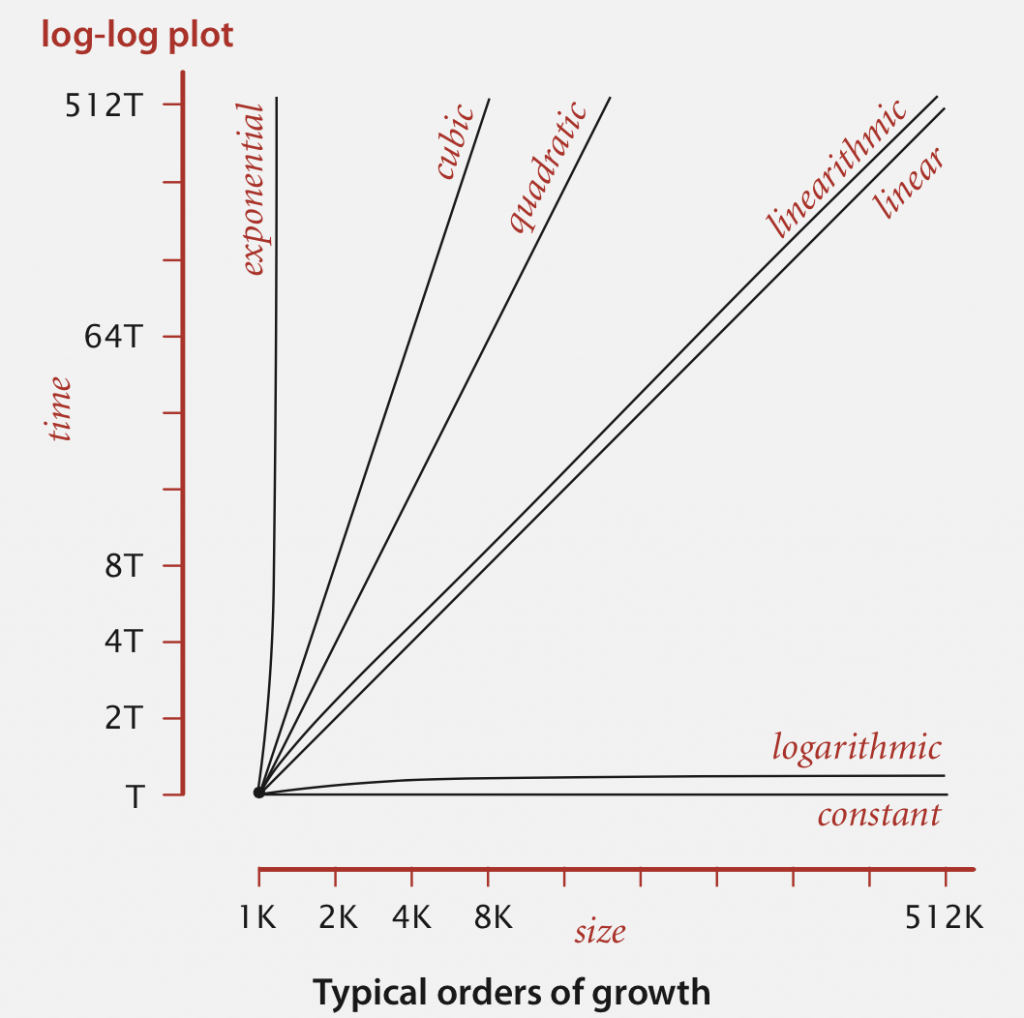And happily, every order has some corresponding code framework: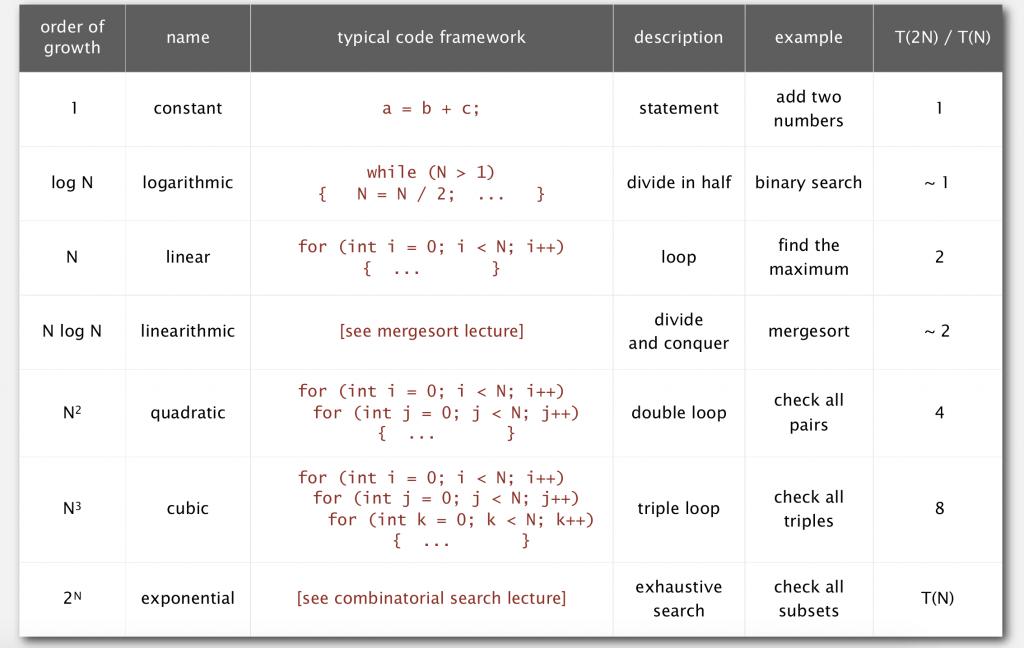Still remember the 3-sum problem? We have 3 for loops in the brute-force version, whose order of growth is N3.

As we can see in the log-log plot, cubic algorithms are hardly good algorithms. In fact,  there is cleverer algorithm for 3-sum problem:

• step1: sort the N numbers
• step: for each pair of numbers a[i] and a[j], binary search for -(a[i]+a[j])

And the order of growth is N2logN(insertion sort and binary search will be covered in future articles):

• step1: N2 for insertion sort
• step2: N2logN with binary search

For N=8000, brute-force version of algorithm takes 51.1 seconds, whereas sort-and-binary-search version takes only 0.96 seconds. This is the magic of algorithm, which charms me into learning more about it.

Better order of growth means faster in practice!

### Theory of algorithms

In theory, there are three types of analyses:

Best cased. Lower bound on cost. Determined by `easiest` input Provides a goal for all inputs

Worst cased. Upper bound on cost. Determined by `most difficult` input Provides a guarantee for all inputs

Average cased. Expected cose for random input Need a model for `random` input Provides a way to predict performance

ur goals are to establish `difficulty` of a problem and develop `optimal` algorithms. When we say `optimal`, we mean the algorithm has performance guarantee for any input and no other algorithms can provide better performance guarantee.

There are some notations commonly used in analysis of algorithm:Let’s take an easy example. To solve 1-sum problem, we have to examine every entry in the array, so the upper bound is O(N), lower bound is Ω(N), and this is the optimal algorithm with running time of Θ(N).

### Memory

Besides running time, another thing we need to keep in mind is how much memory an algorithm needs. Since we use java in this course, we only discuss memory usage in java.

The followng images show how much memory needed for primitive types and objects.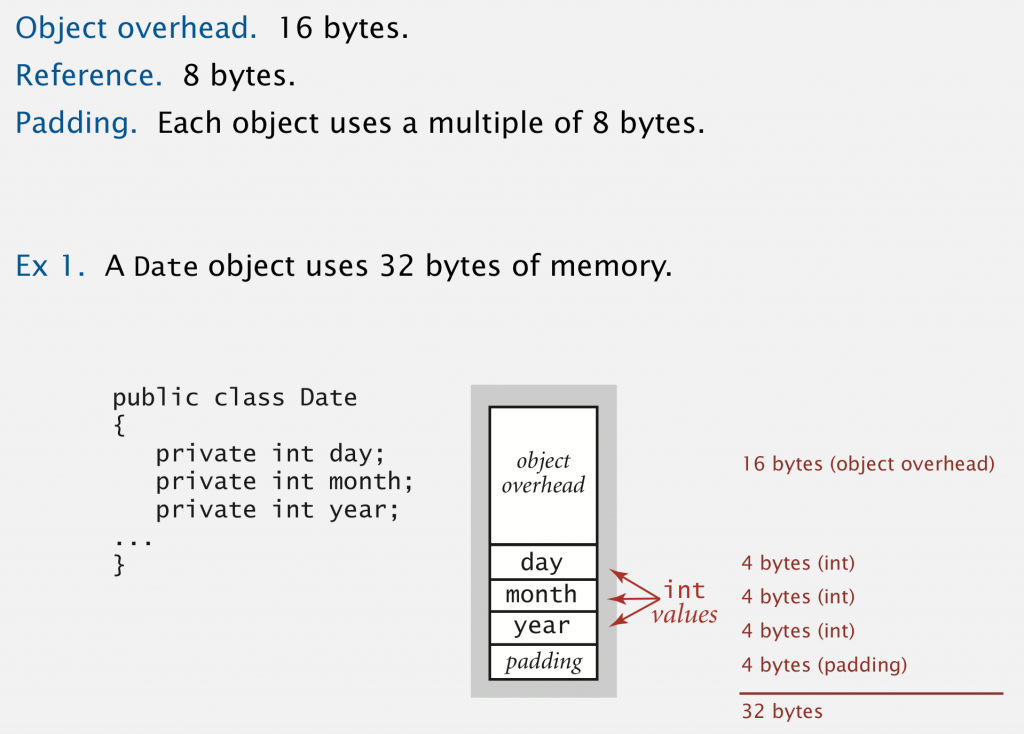With the development of technology, we have larger and larger memory in computers. So this maybe not the most important part to determine whether an algorithm is good or not. But it’s always better if an algorithm can use less memory without changing its order of growth.